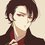# I don't understand

I got this question in a math competition; a multiple choice question:

Suppose $$p$$ is a two-digit number and $$q$$ has the same digits, but in reverse order. The number $$p^2 - q^2$$ is a non-zero perfect square. The sum of the digits of $$p$$ is:

A) 7
B) 9
C) 11
D) 12
E) 13

Can you please solve this problem?Note by Syed Hamza Khalid
1 year, 10 months ago

This discussion board is a place to discuss our Daily Challenges and the math and science related to those challenges. Explanations are more than just a solution — they should explain the steps and thinking strategies that you used to obtain the solution. Comments should further the discussion of math and science.

When posting on Brilliant:

• Use the emojis to react to an explanation, whether you're congratulating a job well done , or just really confused .
• Ask specific questions about the challenge or the steps in somebody's explanation. Well-posed questions can add a lot to the discussion, but posting "I don't understand!" doesn't help anyone.
• Try to contribute something new to the discussion, whether it is an extension, generalization or other idea related to the challenge.

MarkdownAppears as
*italics* or _italics_ italics
**bold** or __bold__ bold
- bulleted- list
• bulleted
• list
1. numbered2. list
1. numbered
2. list
Note: you must add a full line of space before and after lists for them to show up correctly
paragraph 1paragraph 2

paragraph 1

paragraph 2

[example link](https://brilliant.org)example link
> This is a quote
This is a quote
    # I indented these lines
# 4 spaces, and now they show
# up as a code block.

print "hello world"
# I indented these lines
# 4 spaces, and now they show
# up as a code block.

print "hello world"
MathAppears as
Remember to wrap math in $$ ... $$ or $ ... $ to ensure proper formatting.
2 \times 3 $2 \times 3$
2^{34} $2^{34}$
a_{i-1} $a_{i-1}$
\frac{2}{3} $\frac{2}{3}$
\sqrt{2} $\sqrt{2}$
\sum_{i=1}^3 $\sum_{i=1}^3$
\sin \theta $\sin \theta$
\boxed{123} $\boxed{123}$

Sort by:

Let $a$ and $b$ be digits. Now, let $\displaystyle p=10a+b$ and $\displaystyle q=10b+a$.

Substituting these values, we get $\displaystyle p^2-q^2=99\left(a^2-b^2\right)$. And thus, for this to be a perfect square, $\displaystyle a^2-b^2=11$.

Solving these equations give $p=65$ making the answer C.

- 1 year, 10 months ago

What does "Solving these equations" mean? By trial and error? Can you further demonstrate the process?

- 1 year, 10 months ago

Well, we only need to figure out the values of $a$ and $b$ because then $p$ and $q$ will be determined. So for solving $\displaystyle a^2-b^2=11$ observe that this implies

$\left(a-b\right)\left(a+b\right)=11$

Now, the LHS is an integer which implies that either $a-b=11$ and $a+b=1$ or $a-b=1$ and $a+b=11$

We see that only the second pair of equations holds true...….hence it is solved.

- 1 year, 10 months ago

Thanks... I understood this part now but can you tell me how you got from $p^2 - q^2 = 99 (a^2 -b^2)$ to $a^2 -b^2 = 11$.

(I'm sorry to ask you such questions but I am not so good at competition math)

- 1 year, 10 months ago

No need for saying sorry!!! We are all here to learn new stuff!!

According the statement of the question, we need $\displaystyle 3^2\cdot11\left(a^2-b^2\right)$ to be a perfect square. That means $a^2-b^2=11m^2$ for some integer $m$. Now I think you can proceed from here onwards, by checking the possible values of $m$ i.e. $1,2,3$.

- 1 year, 10 months ago

Thanks alot... :)

- 1 year, 10 months ago

Let $p = 10a + b$ where $a, b$ are non-zero digits. Then $q = 10b + a$ and $p^{2} - q^{2} = (10a + b)^{2} - (10b + a)^{2} = 99a^{2} - 99b^{2} = 3^{2} \times 11 \times (a - b)(a + b)$.

For this to equal a non-zero perfect square we require $a \gt b$ and, since $11$ is prime, that (i) $11 | (a + b)$ and (ii) $(a - b)$ is a perfect square. Condition (i) gives us the options for $(a,b)$ of $(9,2), (8,3), (7,4), (6,5)$, but of these only $(a,b) = (6,5)$ satisfies condition (ii). Thus $p = 65$ and indeed $65^{2} - 56^{2} = 1089 = 33^{2}$.

- 1 year, 10 months ago

Let $p=10x+y$ and $q=10y+x$. Then $p^2-q^2=(p+q)(p-q)=(11x+11y)(9x-9y)=(9)(11)(x+y)(x-y)$. Since $x$ and $y$ are non-zero digits, each of them may be $1,2,3,4,5,6,7,8$ or $9$. So $x+y<19$ and $x-y<9$. Also, since $11$ is a prime number, $x+y$ can only be $11$, so that $x-y$ can only be $1$. Therefore $x=6$ and $y=5$. Hence $x+y=11$.

- 1 year, 10 months ago

How did you set up the inequality $x + y < 19$ and $x - y < 9$ ?

- 1 year, 10 months ago

See, x is at most 9 and so also y. So $x+y$ is at most 18. Similarly, both x and y can at least be 1. So $x-y$ is at most $9-1=8$.

- 1 year, 10 months ago

Thanks, can you also tell why $x+ y$ can only be 11 ?

- 1 year, 10 months ago

Because the next larger number is $11*2^2=44$ which is more than $18$

- 1 year, 10 months ago

okay thanks

- 1 year, 10 months ago